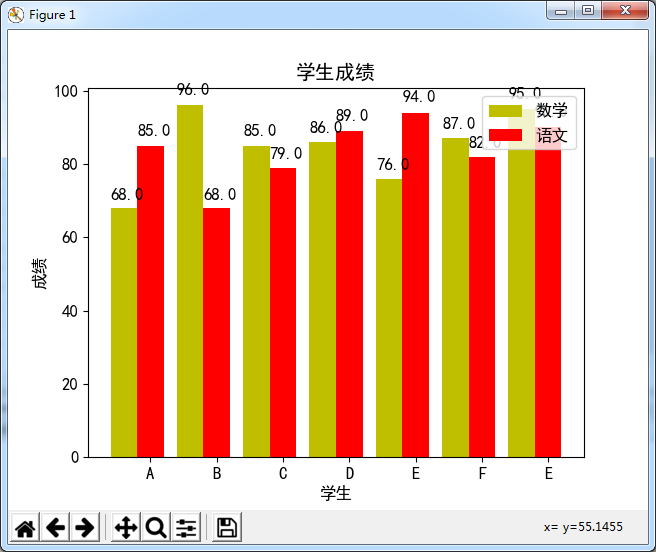# Python实现绘制双柱状图并显示数值功能示例

更新时间：2018年06月23日 02:13:57   作者：水之魂2018我要评论

```# -*- coding:utf-8 -*-
#! python3
import matplotlib.pyplot as plt
import mpl_toolkits.mplot3d
#定义函数来显示柱状上的数值
def autolabel(rects):
for rect in rects:
height = rect.get_height()
plt.text(rect.get_x()+rect.get_width()/2.-0.2, 1.03*height, '%s' % float(height))
if __name__ == '__main__':
l1=[68, 96, 85, 86, 76,87, 95]
l2=[85, 68, 79, 89, 94, 82,90]
name=['A','B','C','D','E','F','E']
total_width, n = 0.8, 2
width = total_width / n
x=[0,1,2,3,4,5,6]
plt.rc('font', family='SimHei', size=12)#设置中文显示，否则出现乱码！
a=plt.bar(x, l1, width=width, label='数学',fc = 'y')
for i in range(len(x)):
x[i] = x[i] + width
b=plt.bar(x, l2, width=width, label='语文',tick_label = name,fc = 'r')
autolabel(a)
autolabel(b)
plt.xlabel('学生')
plt.ylabel('成绩')
plt.title('学生成绩')
plt.legend()
plt.show()

```